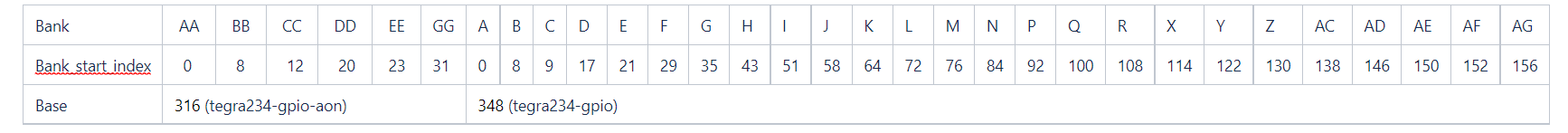# Calculating GPIO Index in Linux

The following section describes how to calculate the GPIO index:

1. The Orin GPIOs are grouped in banks and each bank has up to 8 pins. The bank and the pin information of a GPIO is fundamental for calculating its index.

GPIO is represented as `X.Y`, where:

• X encodes the bank and can have the following values: PA, PB, …, PY or PAA, ... PFF as listed in table below..

For the banks with naming scheme P*, the "P" must be ignored, i.e., "PA" refers to bank A.

• Y encodes the pin within a bank. For example, 00 for pin 0, 01 for pin 1, …, and 07 for pin 7.
2. The banks are associated to a base and can also be sequentially translated into a bank_index as shown in the table below:The base numbering may change in cases where customization is done in kernel and can be identified using the dmesg log:
``````nvidia@tegra-ubuntu:~\$ cat /sys/kernel/debug/gpio | grep gpiochip
[ 9.965908] gpiochip0: registered GPIOs 348 to 511 on tegra234-gpio
[ 9.970024] gpiochip1: registered GPIOs 316 to 347 on tegra234-gpio-aon
``````

The base is 348 for tegra234-gpio and 316 for tegra234-gpio-aon from the above log.

3. The GPIO index can be calculated as: GPIO_index = base + bank_start_index + pin.

The following is an example of calculating the GPIO index for PS.05.

1. PS.05 represents bank S and pin 5.
2. Bank S has bank_index 113 and base 348(tegra-gpio base)
3. GPIO index is 466 (466 = 348 + 113 + 5)